Get instant live expert help with Excel or Google Sheets“My Excelchat expert helped me in less than 20 minutes, saving me what would have been 5 hours of work!”

#### Post your problem and you'll get expert help in seconds

Your message must be at least 40 characters
Our professional experts are available now. Your privacy is guaranteed.

# How to Count Cells that Contain Negative Numbers in Excel

We can count cells that contain a negative number within a specified range of cells with the COUNTIF function. We will walk through the procedure by following the simple steps below.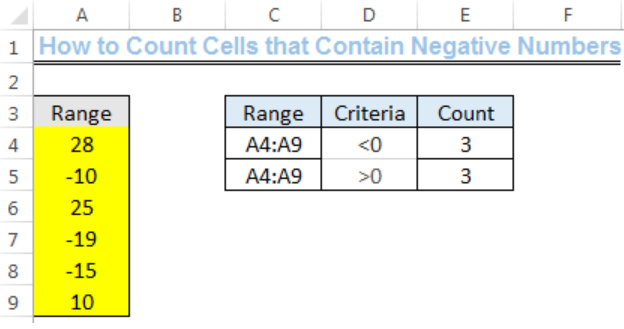Figure 1: COUNTIF Function for counting negative and positive numbers

## Syntax

`=COUNTIF (range, “<0”)`

## Formula

`=COUNTIF(A4:A9,"<0")`

## Setting up the Data

• We will set up our data by inputting the POSITIVE and NEGATIVE numbers into Column A, titled, RANGE.
• We will name Cell C3 as Range, Cell D3 as Criteria, and Cell E3 as Count.
• The Range is A4:A9 and the criteria are for the formula to count cells that have numbers that are greater than and less than zero.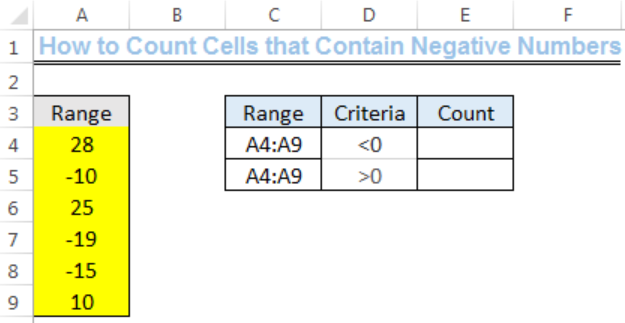Figure 2: COUNTIF Function for counting negative numbers

## Count of Cells that Contain Negative Numbers

We will input the formula below into Cell E4.

`=COUNTIF(A4:A9,"<0")`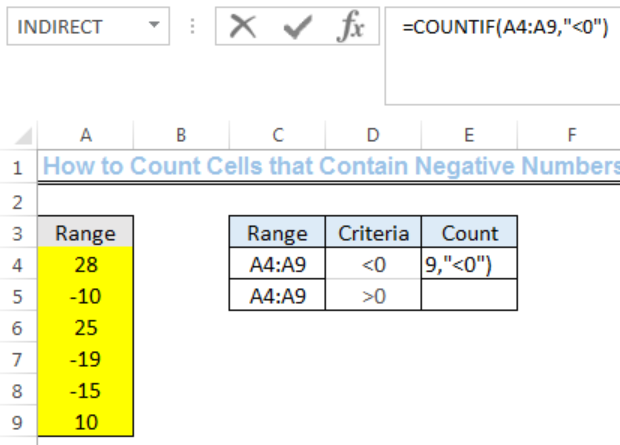Figure 3: COUNTIF Function for counting negative numbers

We will press enter to get the result.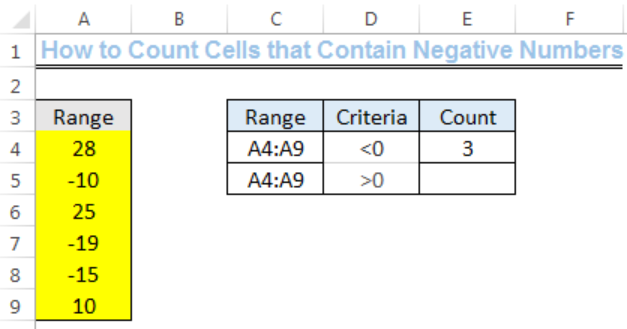Figure 4: COUNTIF Function for counting negative numbers

## Count of Cells that Contain Positive Numbers

We can do this by changing the less than sign to greater than sign. We will input the formula below into Cell E5 and press enter.

`=COUNTIF(A4:A9," >0")`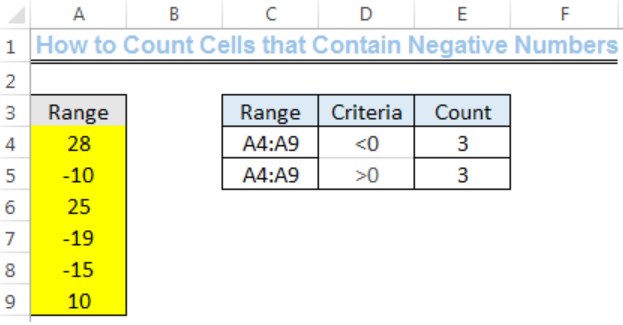Figure 5: COUNTIF Function for counting positive numbers

## Explanation

The COUNTIF function counts the numbers that are present in a range that complies with specified criteria. Where the specified criteria is less than zero, the COUNTIF function returns the number of cells that have values which are less than zero.

## Instant Connection to an Expert through our Excelchat Service

Most of the time, the problem you will need to solve will be more complex than a simple application of a formula or function. If you want to save hours of research and frustration, try our live Excelchat service! Our Excel Experts are available 24/7 to answer any Excel question you may have. We guarantee a connection within 30 seconds and a customized solution within 20 minutes.

### Did this post not answer your question? Get a solution from connecting with the expert.Another blog reader asked this question today on Excelchat:## Subscribe to Excelchat.coAnother blog reader asked this question today on Excelchat: